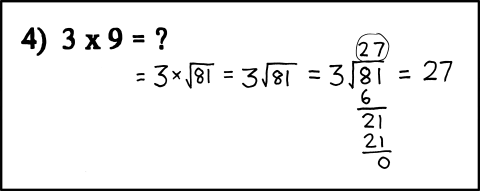← Back

June 28, 2010

# #759: 3x9[[A problem is given on an arithmetic test: “4) 3x9=?”. In handwriting, the student’s work follows. The student has accurately reformatted the question as 3 times the square root of 81, which visually resembles the long division problem of 3 divided into 81, and then solved the latter to get 27–the correct answer to both.]]